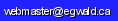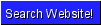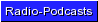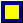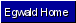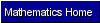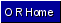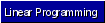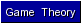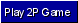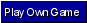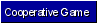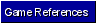Operations Research - Game Theory

by

Elmer G. Wiens

Egwald's popular web pages are provided without cost to users.
Please show your support by joining Egwald Web Services as a Facebook Fan:Follow Elmer Wiens on Twitter:Game Theory - Introduction | Battle of the Sexes | Prisoner's Dilemma | Free Rider | Game of Chicken | Play a Game Online | Metagame Example

Cooperative Two Person Generalized (Non-Zero) Sum Games

Battle of the Sexes

Zero sum games assume that one player's gain is the other player's loss. Fortunately, interpersonal contexts exist where players, through cooperation, can obtain outcomes preferred by both players. Games that model such situations are called negotiated games.

If the players agree to coordinate their strategies, the resulting payoff to the players is called a negotiated settlement.

Consider the classical model of "The Battle of the Sexes" found in Anatol Rapoport.

He and she want to meet for the evening, but cannot agree on which of two events to attend. He prefers a baseball game; she prefers the theatre. Both prefer going out together to going to either event by themselves. In the payoff matrix below, the first (second) entry in each cell of the first row (column) represents the pay-off to Man (Woman) of going to the baseball game. The first (second) entry in each cell of the second row (column) represents the payoff to Man (Woman) of going to the theatre. These payoffs are, of course, conditional on the choice of the other player.

 Game Tableau Woman baseball theatre Y1 Y2 Man baseball X1 1, 0 -a, -b theatre X2 -c, -d 0, 1

Certain restrictions apply: 0 < a,b,c,d < 1, and min(a, b) > max(c,d).

X1 is the probability that Man attends the baseball game; X2 is the probability that Man attends the theatre; X2 = 1 - X1; Y1 is the probability that Woman attends the baseball game; Y2 is the probability that Woman attends the theatre; Y2 = 1 - Y1.

The worst that can happen is that they fail to agree. Woman goes to the theatre; Man goes to the baseball game. Their joint payoff is (-a, -b). This outcome is sometimes called a threat point: it is the outcome that obtains if each player is selfish.

Also bad is if each player insists on the preferred event of the other player. Woman goes to the ball game expecting to satisfy Man; Man goes to the theatre. Now their joint payoff is (-c, -d). The restrictions on the coefficients ensure that misplaced altruism is better than selfishness. Both players feel slightly better, even though they are not together, because they took the other's preferences into consideration.

If Woman and Man expect to got out frequently, they could agree to alternate going together to the ball game and theatre. Their expected payoff would be (.5, .5). If it is just a one-night stand, they could draw straws.

However, if the game is not symmetric in terms of payoffs, say if a > b, then the greater loss to Man of the (baseball, theatre) outcome should be reflected in the proportion of times they go to the baseball game — the probability of going to the baseball game.

Whatever the proportion of times they go to either event together, the outcome can be represented by the coordinates of a point on the line in red joining the red points (1, 0) and (0,1) in the diagram below. In this diagram the horizontal axis represents Man's payoff; the vertical axis represents Woman's payoff. The blue points are the off-diagonal outcomes of the game matrix. The red line is called the negotiation set; its equation is of course:

Y = f(X) = 1 - X

where X is the probability they attend the ball game

and   Y is the probability they attend the theatre.

The joint payoff to the players on the negotiated set is (X, Y).

The feasible payoff region is the convex set enclosed by the lines joining the payoff pairs.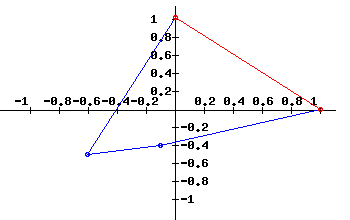Since 1*X + Y = 1 on the negotiation set, the rate at which Man's payoff can be traded for Woman's is 1 — the coefficient of X — called the payoff trade-off rate.

It is easier to work with numbers, rather than letters. Consider the cooperative game below, which is the one in the diagram above.

 Game Tableau Woman baseball theatre Y1 Y2 Man baseball X1 1, 0 -.6, -.5 theatre X2 -.1, -.4 0, 1

The following solutions to cooperative two person games are called Nash solutions - based on the work of John F. Nash.

Solution I.

We can compute "a solution" using the threat point (-.6, -.5) with the Nash Function. Nash showed that if the cooperative game satisfies some reasonable axioms, then the Nash function yields a negotiated settlement based on the given threat point.

1.         G(X) = [X - (-.6)] [f(X) - (-.5)] = [X + .6] [1 - X + .5]

Roughly, G(X) is the product of the distance each player's negotiated payoff is from the threat payoff, taking the payoff trade-off rate into consideration.

This function has a maximum at X = .45. (Set the derivative of G equal to zero and solve for X).

So, the negotiated settlement is (.45, .55). They agree to attend the theatre 55% of the time and the ball game 45% of the time. The higher payoff to Woman reflects her greater threat.

Solution II (Shapley).

Suppose that Man and Woman agree to attend the events together if they happen to meet at the event. How should they play? Man ignores Woman's payoffs and plays a two person zero sum game on his own payoff matrix (called Man's max-min strategy). Its solution is:

 Man'sGame Woman .353 .647 Man .059 1 -.6 .941 -.1 0

where .059 is the probability that the man goes to the baseball game and .941 is the probability that he goes to the theatre. The expected payoff to Man of -0.035 is called Man's security level.

Similarly, Woman ignores Man's payoffs and plays a two person zero sum game on her own payoff matrix (her max-min strategy.). Its solution is:

 Woman'sGame Woman .789 .211 Man .737 0 -.5 .263 -.4 1

with an expected payoff to Woman of -0.105, Woman's security level.

The joint security level of (-.035, -.105) is preferable to the payoff (-.6, -.5) they get if Man always goes to the baseball game and Woman always goes to the theatre.

With the new threat point of (-.035, -.105) the Nash function is:

2.         G(X) = [X - (-.035)] [f(X) - (-.105)] = [X + .035] [1 - X + .105]

with a maximum at X = .535. With security levels as the threat point, the negotiated settlement is (.535, .465), an improvement for Man from solution I.

Man's situation has improved because "meeting by chance" yields a lower expected payoff to Woman than to Man.

Solution III. (Nash)

Clearly, there are some easy objections to solution II. Man is threatening to go to the ball game just 5.9% of the time. Why doesn't Woman, knowing this, always go to the theatre, because Man will be there 94.1% of the time. Then, of course, Man should do something else: he could play (X1 = .737, X2 = .263), his solution from Woman's own game above, holding Woman's expected pay-off to her security level (called Man's min-max strategy).

Nash suggested that Man and Woman use probabilities of attending either event — called threat strategies — that maximize each person's share of the negotiated settlement as determined by the Nash function. These "optimal" threat strategies are calculated from the following two person zero sum game.

This game tableau shows the difference between Man's pay-off and Woman's payoff (in this example Woman's payoff plus Man's payoff equals 1 on the negotiated set). If Man attempts to maximize the expected difference in payoffs, he must solve this game. Woman attempting to maximize the expected difference between her payoff and Man's payoff is equivalent to Woman minimizing the expected payoff of this game.

 Man-WomanPayoff Woman 0 1 Man 1 1 -.1 0 .3 -1

Solving the two person zero sum game in this situation yields optimal pure threat strategies: Man goes to the baseball game and Woman goes to the theatre.

In this case we are back where we started. Man must yield to Woman's superior bargaining position, with a negotiated settlement of (.45, 55).

Without the restrictions 0 < a, b, c, d < 1 placed on the game coefficients, the Nash two person zero sum game based on the differences in payoffs can yield optimal mixed threat strategies, i.e. player's choose each option with some positive probability.

Suppose Man and Woman discover they share a favourite restaurant. After talking through their feelings (payoffs) about going to the ball game, the restaurant, or the theatre, the following game tableau seems appropriate:

 Game Tableau Woman baseball restaurant theatre Y1 Y2 Y3 Man baseball X1 1, 0 0,0 -.6, -.5 restaurant X2 -.2, -.2 .4, .9 0, 0 theatre X3 -.1, -.4 -.2,-.2 0, 1

The new payoff set is displayed below. The negotiated set is a pair of red lines intersecting at the payoff (.4, .9). Man's payoff axis is labelled u; Woman's payoff axis v.The line from (0, 1) to (.4, .9) has the equation .25*u + v = 1.

The line from (.4, .9) to (1, 0) has the equation 1.5*u + v = 1.5.

Without the restaurant option, Man already has a payoff of .45. Therefore, with the restaurant option his new payoff should be greater than .45. Therefore, only the line from (.4, .9) to (1, 0) seems relevant.

Along this line (v = 1.5 - 1.5*u) the payoff tradeoff rate is 1.5. Increasing Man's payoff by .1 unit results in a .15 reduction of Woman's payoff.

Let M be the matrix of Man's payoffs; W the matrix of Woman's payoffs. It is known (see Thomas) that optimal threat strategies can be found by solving the zero sum game obtained from the matrix:

1.5 * M - W

 Game Tableau Woman baseball restaurant theatre Y1 Y2 Y3 Man baseball X1 1.5 0 -0.4 restaurant X2 -0.1 0.3 0 theatre X3 0.25 -0.1 -1

Solving the game yields the optimal mixed threat strategies and payoffs if the threats are played on matrices M and W;

X1 = 0.05; X2 = 0.95; X3 = 0; payoff u = -0.052.

Y1 = 0.2; Y2 = 0; Y3 = 0.8; payoff v = -0.0605.

The threats suggest that Man will go to the ball game 5% of the time; the restaurant 95% of the time. Woman will go to the ball game 20% of the time; the theatre 80% of the time. They only meet by chance at the ball game 1% of the time (.01 = .05 * .2).

With the new threat point of (-.052, -.0605) the Nash function is:

2.         G(u) = [u - (-.052)] [1.5 - 1.5*u - (-.0605)]

The Nash function has a maximum at u = 0.494, implying v = 1.5 - 1.5u = 0.759.

Using a bit of trigonometry, the length of the line from (1, 0) to (.4, .9) is 1.08173; the length of the line from (1, 0) to (.494, .759) is 0.9119. The negotiated settlement is 84.3% of the distance from (1, 0) along the negotiated set. Thus, (.494, .759) = .843 * (.4, .9) + .157 * (1, 0).

The negotiated settlement has our couple going — together — to the restaurant 84.3% of the time; the ball game 15.7% of the time. The joint payoff is:

(Man, Woman) = (.494, .759) — an improvement in both payoffs from the original game.

Nash's Axioms

1. Player Symmetry: Switching the names and payoffs of players doesn't matter.
2. Linear Transformations: Taking a linear transformation of a player's payoffs doesn't matter.
3. Independence of Irrelevant Alternatives: Adding more cells to the payoff matrix doesn't count unless the new cell is part of the new solution.
4. Pareto-Optimality: If players can negotiate a settlement that is better for both of them than the status quo, then they will do so.
5. Individual Rationality: Given a choice, a player will choose the option with the highest payoff.

Other Solutions

Solving cooperative games is a game in itself. Many solutions exist. For examples see Braithwaite or Raiffa.

References

• Braithwaite, R. B. Theory of Games as a Tool for the Moral Philosopher. Cambridge: Cambridge UP, 1955.
• Nash, John F. "The Bargaining Problem." Econometrica. 18 (1950): 155-162.
• Owen, Guillermo. Game Theory, 2nd Edition. Academic: New York, 1982.
• Rapoport, Anatol. Two-Person Game Theory: The Essential Ideas. Ann Arbor: U Michigan: 1966.
• Raiffa, H. "Arbitration Games for Generalized Two-Person Games." Contributions to the Theory of Games, II (Annals of Mathematics Studies, 28). Ed. Kuhn, H. W. and A. W. Tucker, Princeton, N.J.: Princeton UP, 1953.
• Shapley, L. S. "A Value for N-Person Games." Contributions to the Theory of Games, II (Annals of Mathematics Studies, 28). Ed. Kuhn, H. W. and A. W. Tucker, Princeton, N.J.: Princeton UP, 1953.
• Thomas, L. C. Games, Theory and Applications. New York: John Wiley, 1984.Copyright © Elmer G. Wiens:   Egwald Web ServicesAll Rights Reserved.    Inquiries